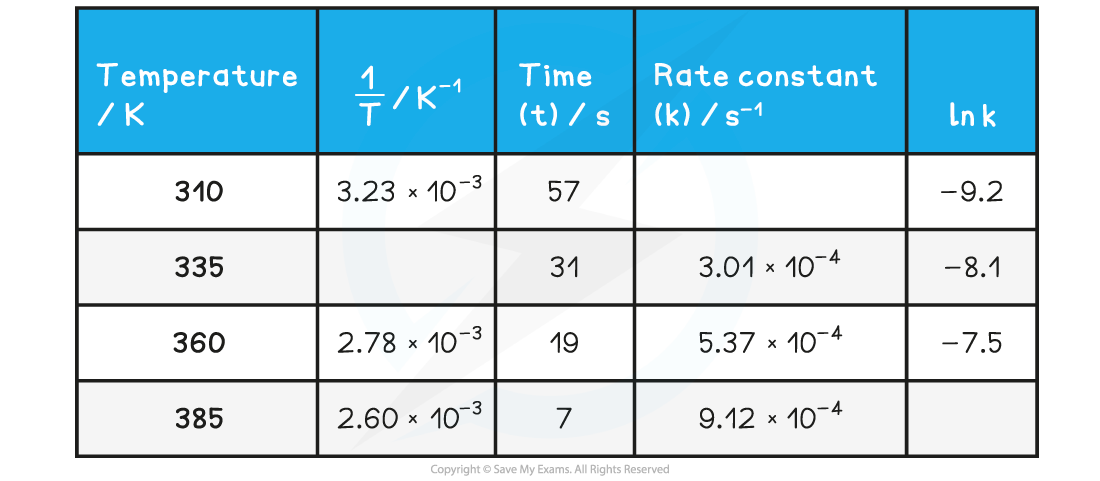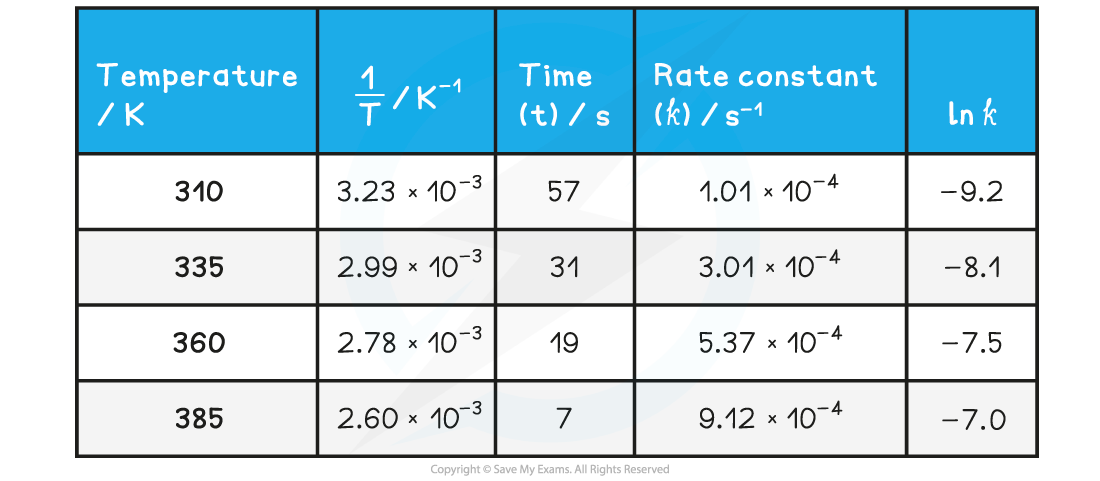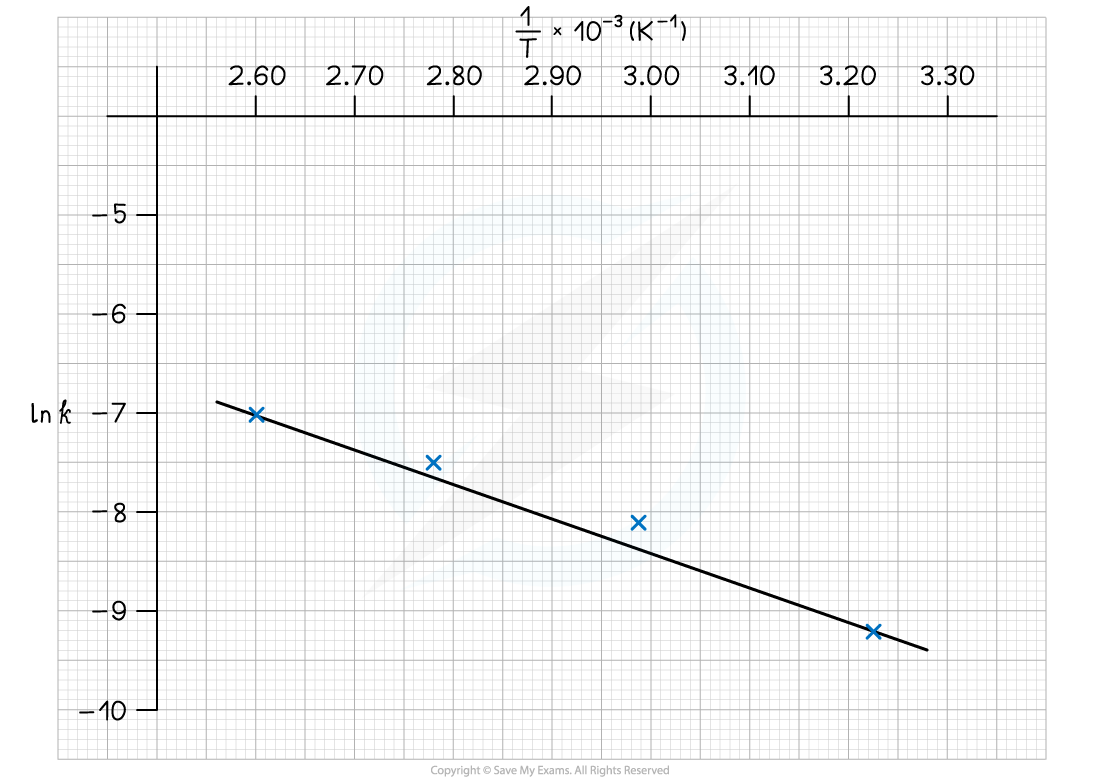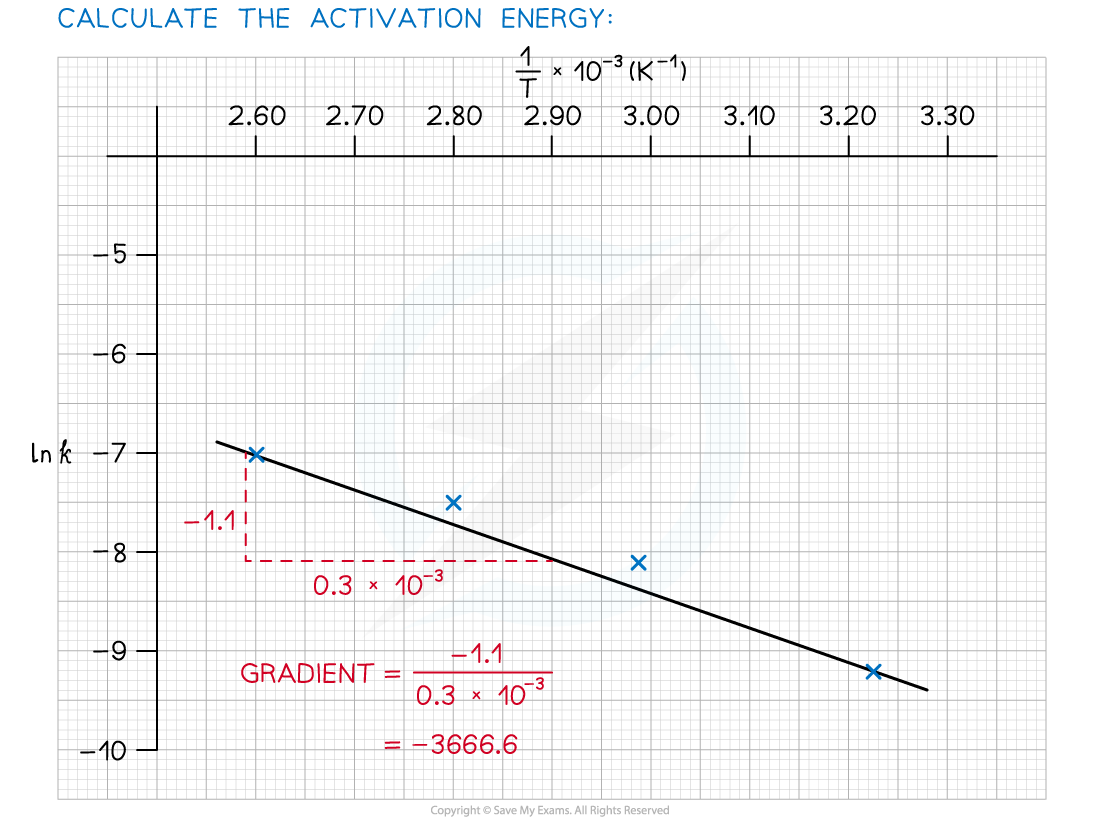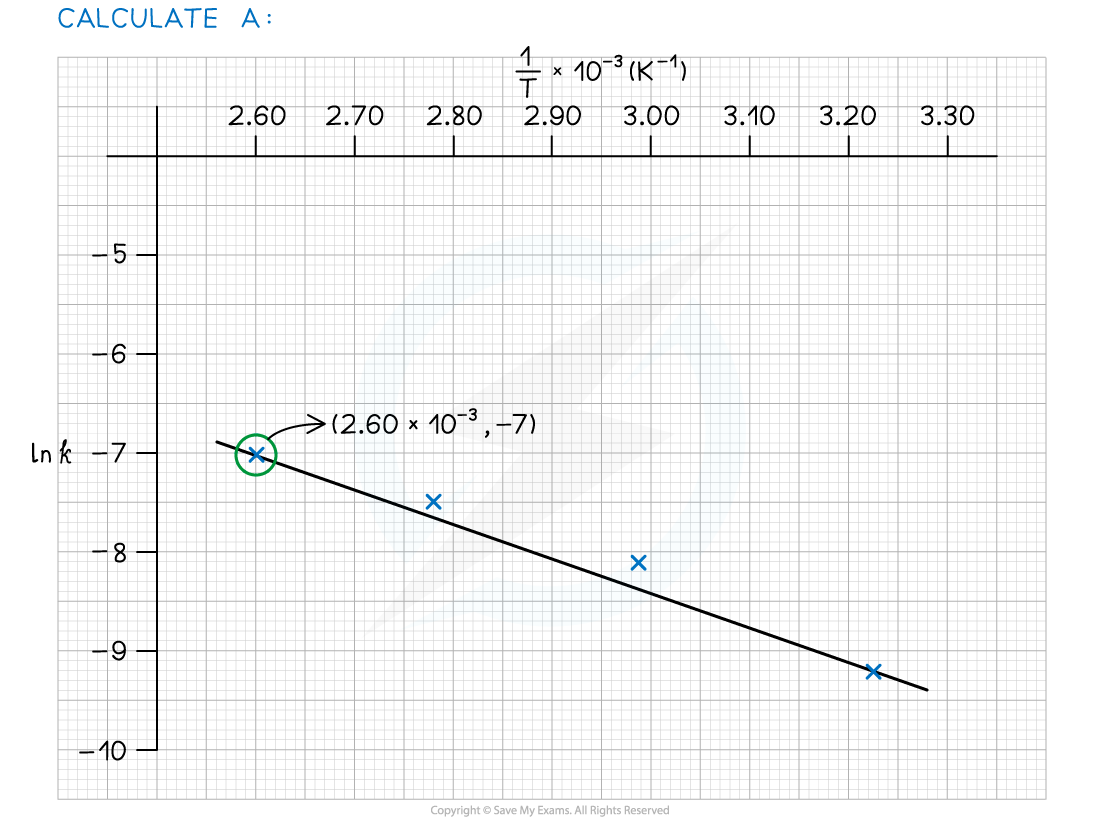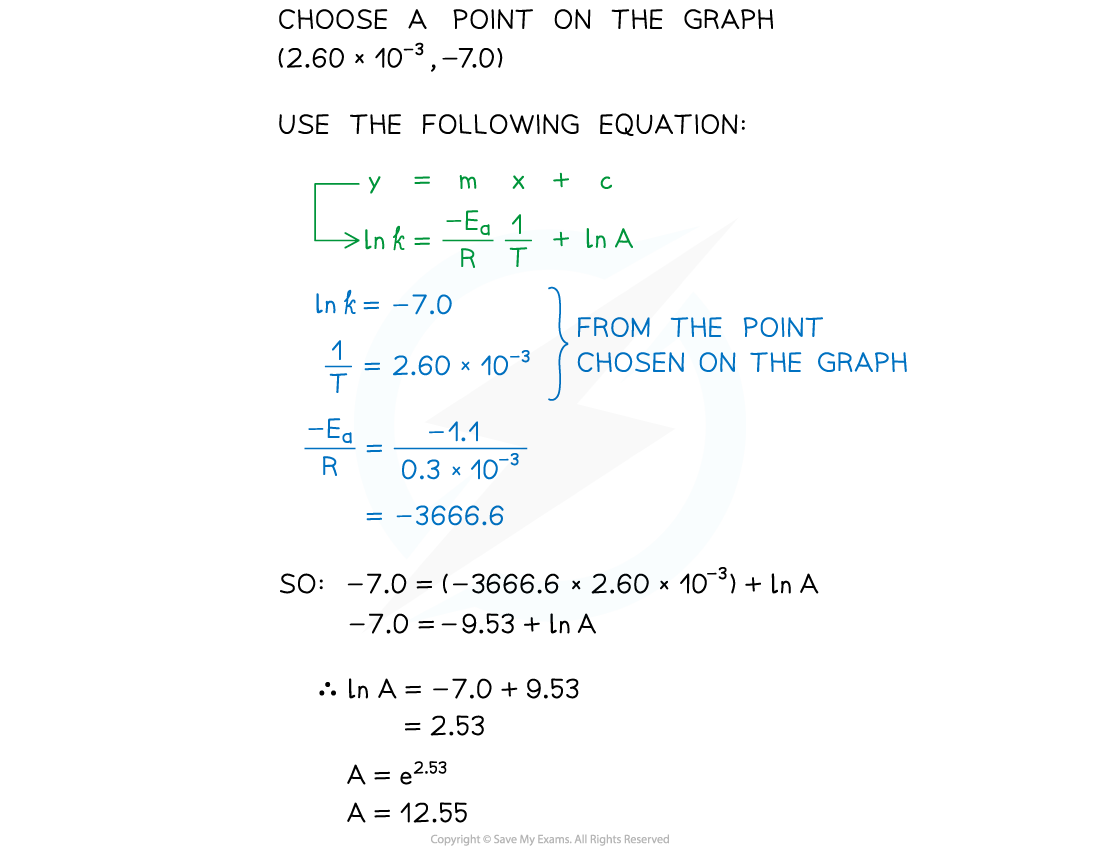# Edexcel A Level Chemistry:复习笔记5.5.9 Arrhenius & Activation Energy

### Arrhenius & Activation Energy

• The rate equation shows how each of the reactants in a reaction effects the rate of the reaction and it includes the rate constant, k
• However, k only remains constant if the concentration of the reactants is the only factor which is changed
• If the temperature is changed or a catalyst is used or changed, then the rate constant, k, changes

• At higher temperatures, a greater proportion of molecules have energy greater than than the activation energy
• Since the rate constant and rate of reaction are directly proportional to the fraction of molecules with energy equal or greater than the activation energy, then at higher temperatures:
• The rate of reaction increases
• The rate constant increases

• The relationship between the rate constant, the temperature and also the activation energy is given by the Arrhenius equation: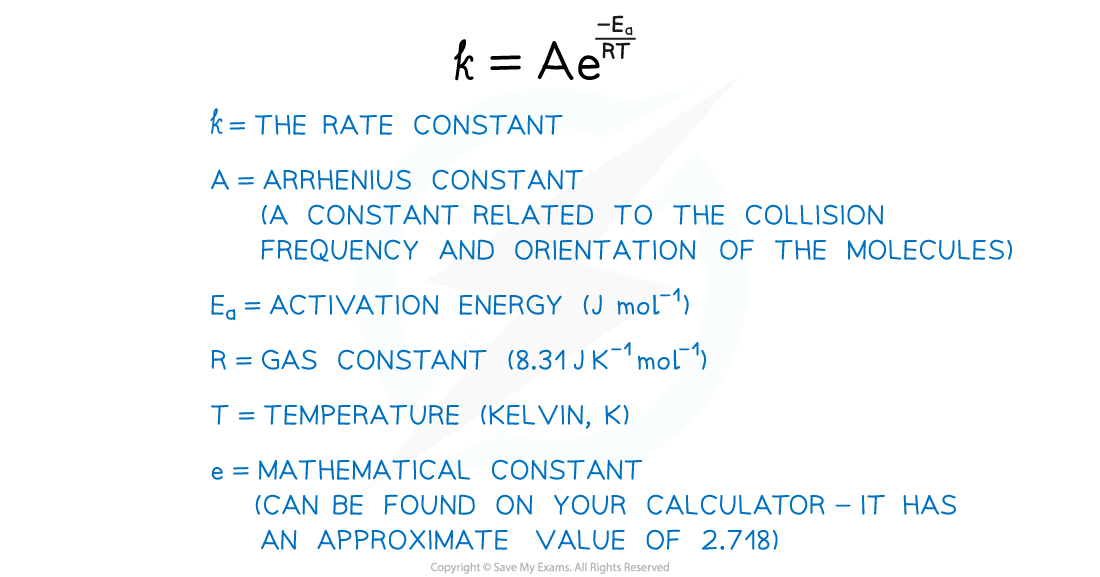• Ea and A are constants that are characteristic of a specific reaction
• A does vary slightly with temperature but it can still be considered a constant

• R is a fundamental physical constant for all reactions
• k and T are the only variables in the Arrhenius equation

• The Arrhenius equation is used to describe reactions that involve gases, reactions occurring in solution or reactions that occur on the surface of a catalyst

#### Finding the Activation Energy

• Very often, the Arrhenius equation is used to calculate the activation energy of a reaction
• A question will either give sufficient information for the Arrhenius equation to be used or a graph can be plotted and the calculation done from the plot

#### Using the Arrhenius Equation

• The Arrhenius equation is easier to use if you take natural logarithms of each side of the equation, which results in the following equation:• The Arrhenius Equation can be used to show the effect that a change in temperature has on the rate constant, k, and thus on the overall rate of the reaction
• An increase in temperature (higher value of T) gives a greater value of ln k and therefore a higher value of k
• Since the rate of the reaction depends on the rate constant, k, an increase in k also means an increased rate of reaction

• The equation can also be used to show the effect of increasing the activation energy on the value of the rate constant, k
• An increase in the activation energy, Ea, means that the proportion of molecules which possess at least the activation energy is less
• This means that the rate of the reaction, and therefore the value of k, will decrease

• The values of k and T for a reaction can be determined experimentally
• These values of k and T can then be used to calculate the activation energy for a reaction
• This is the most common type of calculation you will be asked to do on this topic

#### Worked Example

Calculate the activation energy of a reaction which takes place at 400 K, where the rate constant of the reaction is 6.25 x 10-4 s-1.

A = 4.6 x 1013 and R = 8.31 J mol-1 K-1.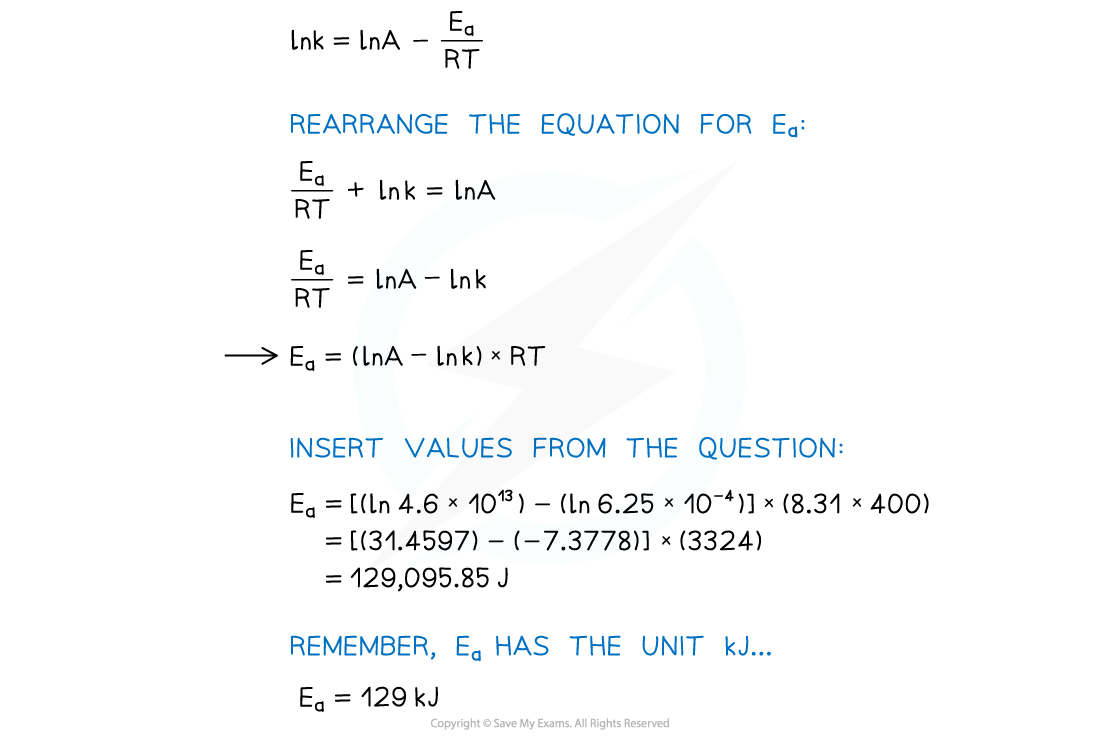#### Using an Arrhenius plot:

• A graph of ln k against 1/T can be plotted, and then used to calculate Ea
• This gives a line which follows the form y = mx + c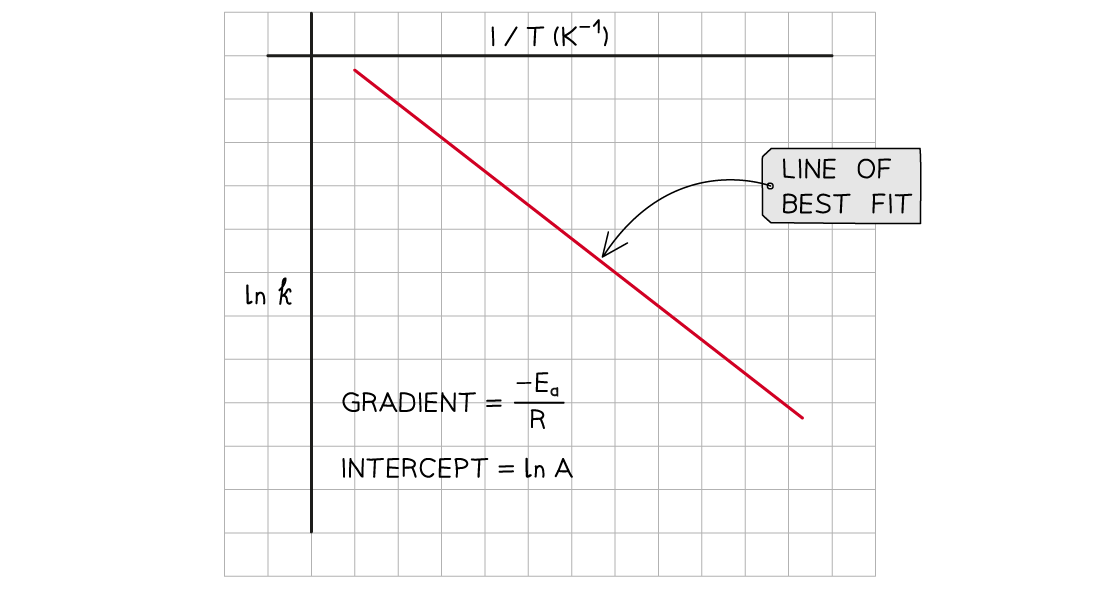The graph of ln k against 1/T is a straight line with gradient -Ea/R

• From the graph, the equation in the form of y = mx + c is as follows:#### Worked Example

1. Complete the following table
2. Plot a graph of ln k against 1/T
3. Use this to calculate the activation energy, Ea, and the Arrhenius constant, A, of the reaction.